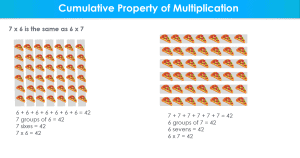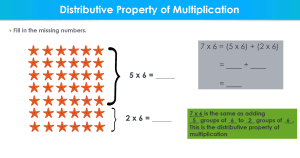Math Activities

# How To Use A Multiplication Array

One of the questions asked today is how to use a multiplication array to help your child master the multiplication tables. Using this model isn’t necessarily new and you may even remember using some form of it in your early years of education. Same as then, the arrangement of rows and columns used to solve multiplication problems can be duplicated in many ways. Whether you use hands-on manipulatives or draw pictures on a piece of paper, this concept can help your child master their multiplication facts.

## What is a Multiplication Array?A multiplication array is an arrangement of columns or rows that match a multiplication equation. You can see examples of arrays in everyday life from the way rows (and products) are laid out in the grocery store to the way sets are laid out in a movie theatre.

When it comes to a multiplication equation, you can create an array using a number of things. Some of the easiest to create are using dots arranged in the matching rows and columns to match the multiplication equation. You can also use physical objects such as small rocks or colored dots.

## How to Use the Multiplication Array To Teach the Times TableOne of the easiest ways to use the multiplication array to teach the times table is by using manipulatives. For younger children, having hands-on objects that they can manipulate will help their brains work even more to solve the problem. They can also use objects written or drawn on a piece of paper.

First and foremost, children will need to understand what an array is and make the connection between what it is and how to use them. Using an everyday example would be a good place to start. For example, you could highlight how things are shelved and placed at grocery stores. You can also use how chairs are placed in an auditorium.

Then, you can begin to introduce different multiplication facts for them to use an array to solve. If there aren’t any objects that can be used, have your child draw circles on a piece of paper. Give them a multiplication problem such as 4×6 and have them illustrate it on their paper using an array of circles.

Then, switch things up by giving them a multiplication problem in array form and have them write out the problem and answer numerically. Either of these formats will help your child become more fluid in working and solving multiplication problems.

## How to use a Multiplication Array to teach the properties of multiplication

Having a sound understanding of number properties can make doing any type of mathematics much easier. Young children are introduced to several properties even if they are not familiar with their proper names.

### Cumulative Property of Multiplication

The cumulative property states that changing the order of numbers in a number sentence does not change the answer. 7 x 6 is the same as 6 x 7. Using an array can better help a child understand this concept. When a child can apply this property while working on multiplication problems, they will not have to memorize facts. They’ll instantly know that 2×6 will yield the same answer as 6×2.

###### Distributive Property of Multiplication

This property basically takes an array and helps children use an “add-on” frame of thinking to reach the answer of a multiplication problem. For example, 7 x 6 is the same as adding 5 x 6 and 2 x 6. Using an array, a student can add on the correct number of “sets” needed to reach the desired answer of a problem.

##### Division as the Inverse Operation of Multiplication

After reaching a certain level in math, children begin to make connections that addition and subtraction work hand-in-hand as do multiplication and division. Oddly though, division is one of the hardest concepts for children to master. Thanks to using a multiplication array, children can see the correlation between multiplication and division, thus making it easier to work those problems as well.

## Benefits of Using a Multiplication Array to Teach Multiplication

### Using a multiplication array helps children see the multiplication problems more clearly.

There are some children who can see a problem in their mind and work it out that way. However, that’s not the case for most children. When they can see a problem by using an array, it becomes easier to understand how to work it.

### Multiplication arrays help children understand how to use strategy.

In most classrooms (and homeschools), you’ll find mathematics taught in a rote memorization kind of format. Teachers and parents will typically use some form of flashcards and/or copy work to get students to “memorize facts.” The primary issue with this is when a child is met with a problem they need to work out, they usually struggle with finding the answer.

By using multiplication arrays, children can begin to see patterns and use stepping stones to solve problems.

## Conclusion

If you’ve been looking for practical ways to use a multiplication array to help your child master the multiplication tables, the examples above will help you do it. Overall, it’s more about tapping into what’s already going on in the minds of children. Start by gathering some hands-on manipulatives, then slowly introducing arrays with small multiplication problems. Then, gradually add more.